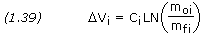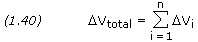## Staging

Rocket Propulsion:

– Thrust

– Conservation of Momentum

– Impulse & Momentum

– Combustion & Exhaust Velocity

– Specific Impulse

– Rocket Engines

– Power Cycles

– Engine Cooling

– Solid Rocket Motors

– Monopropellant Engines

– Staging

A multi stage rocket is a rocket with two or more stages, each with its own engine and propellant, to travel through space.The result is two or more separate rockets, attached either on top or next two each other.The first stage is at the bottom and is usually the largest, the second stage and subsequent upper stages are above it, usually decreasing in size.Once fuel is used up in a certain stage of the rocket, that stage can be dropped, making the rocket lighter. The lighter the rocket, the more fuel efficient and faster it can go. Also, each stage can have a different motor specific to the area it would be traveling through at the time the motor will need to be used.

Staging makes the motors not being used until later in flight and the entire rocket more difficult to build. In addition, each staging event has a greater probability of something going wrong. There could be separation failure, ignition failure, or stage collision as well as normal rocket failures.

Multistage rockets allow improved payload capability for vehicles with a highV requirement such as launch vehicles or interplanetary spacecraft. In a multistage rocket, propellant is stored in smaller, separate tanks rather than a larger single tank as in a single-stage rocket. Since each tank is discarded when empty, energy is not expended to accelerate the empty tanks, so a higher totalV is obtained. Alternatively, a larger payload mass can be accelerated to the same totalV. For convenience, the separate tanks are usually bundled with their own engines, with each discardable unit called a stage.

Multistage rocket performance is described by the same rocket equation as single-stage rockets, but must be determined on a stage-by-stage basis. The velocity increment,Vi, for each stage is calculated as before,where moi represents the total vehicle mass when stage i is ignited, and mfi is the total vehicle mass when stage i is burned out but not yet discarded. It is important to realize that the payload mass for any stage consists of the mass of all subsequent stages plus the ultimate payload itself. The velocity increment for the vehicle is then the sum of those for the individual stages where n is the total number of stages.We define the payload fraction as the ratio of payload mass to initial mass, or mpl/mo.

For a multistage vehicle with dissimilar stages, the overall vehicle payload fraction depends on how theV requirement is partitioned among stages. Payload fractions will be reduced if theV is partitioned suboptimally. The optimal distribution may be determined by trial and error. AV distribution is postulated and the resulting payload fraction calculated. TheV distribution is varied until the payload fraction is maximized. Once theV distribution is selected, vehicle sizing is accomplished by starting with the uppermost or final stage (whose payload is the actual deliverable payload) and calculating the initial mass of this assembly. This assembly then forms the payload for the previous stage and the process repeats until all stages are sized. Results reveal that to maximize payload fraction for a givenV requirement:

1. Stages with higher Isp should be above stages with lower Isp.
2. MoreV should be provided by the stages with the higher Isp.
3. Each succeeding stage should be smaller than its predecessor.
4. Similar stages should provide the sameV.

```PROBLEM 1.12

A two-stage rocket has the following masses:  1st-stage propellant mass 120,000
kg, 1st-stage dry mass 9,000 kg, 2nd-stage propellant mass 30,000 kg, 2nd-stage
dry mass 3,000 kg, and payload mass 3,000 kg.  The specific impulses of the
1st and 2nd stages are 260 s and 320 s respectively.  Calculate the rocket's
totalV.

SOLUTION,

Given:  Mo1 = 120,000 + 9,000 + 30,000 + 3,000 + 3,000 = 165,000 kg
Mf1 = 9,000 + 30,000 + 3,000 + 3,000 = 45,000 kg
Isp1 = 260 s
Mo2 = 30,000 + 3,000 + 3,000 = 36,000 kg
Mf2 = 3,000 + 3,000 = 6,000 kg
Isp2 = 320 s

Equation (1.24),

C1 = Isp1g
C1 = 260 × 9.80665 = 2,550 m/s

C2 = Isp2g
C2 = 320 × 9.80665 = 3,138 m/s

Equation (1.39),V1 = C1 × LN[ Mo1 / Mf1 ]V1 = 2,550 × LN[ 165,000 / 45,000 ]V1 = 3,313 m/sV2 = C2 × LN[ Mo2 / Mf2 ]V2 = 3,138 × LN[ 36,000 / 6,000 ]V2 = 5,623 m/s

Equation (1.40),VTotal =V1 +V2VTotal = 3,313 + 5,623VTotal = 8,936 m/s```

This entry was posted in Rocket Propulsion and tagged , , . Bookmark the permalink.